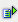The function mod (num, divisor) returns the rest of the division of a value (num) by the divisor (divisor).

Syntax: =mod(num,divisor)Example =mod(0.3) is 0 =mod(1.3) is 1 =mod(2.3) is 2 =mod(3.3) is 0 =mod(4,3) is 1